# MA.912.GR.6.1Export Print
Solve mathematical and real-world problems involving the length of a secant, tangent, segment or chord in a given circle.

### Clarifications

Clarification 1: Problems include relationships between two chords; two secants; a secant and a tangent; and the length of the tangent from a point to a circle.
General Information
Subject Area: Mathematics (B.E.S.T.)
Strand: Geometric Reasoning
Status: State Board Approved

## Benchmark Instructional Guide

• Circle

### Vertical Alignment

Previous Benchmarks

Next Benchmarks

### Purpose and Instructional Strategies

In middle grades, students learned about circles, including the definition of center, radius (radii), diameter and circumference, and they used the Pythagorean Theorem to find lengths of segments. In Algebra 1, students rearranged formulas to highlight a quantity of interest and solved linear and quadratic equations in one variable. In Geometry, students learn about the lengths and relationships between a variety of line segments involving circles.
• Instruction includes using precise definitions and language when working with segments involving circles. Students should be able to determine the similarities and differences between each of the various segments and how their relationships interact with one another. (MTR.4.1
• For example, students should be able to answer questions like “What is the difference between chord and diameter?”, “Is a diameter always a chord?” and “What is the difference between tangent and secant?”
• Instruction includes the understanding that while tangents and secants are often defined as lines, when determining lengths of these, one is referring to just a segment of the tangent or secant. The endpoints of these segments are typically a point of intersection between two lines, a point of intersection between a line and the circle, or a given point that is external to the circle. (MTR.4.1
• Instruction includes the connection to similarity criteria and the Inscribed Angle Theorem to prove, and understand, the relationship between two chords. Typically, the theorem that describes the relationship between two lengths of chords is called the Intersecting Chords Theorem or the Chord-Chord Theorem.
• For example, given the circle shown below with chords AD and CB, two triangles are formed, ΔAPB and ΔCPD. Students should notice that angle APB and angle CPD are congruent because they are vertical angles. Students should also notice that angle ABC and angle ADC are congruent because they intercept the same arc on the circle. Therefore, using the Angle-Angle criterion, students can prove that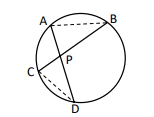• these two triangles are similar. Since corresponding sides are proportional, students can determine that $\frac{\text{AP}}{\text{CP}}$ = $\frac{\text{BP}}{\text{DP}}$. Using this fact, students can rearrange the formula to state that AP ⋅ DP = CP ⋅ BP, which is the Intersecting Chords Theorem.
• Instruction includes the connection to similarity criteria and the Inscribed Angle Theorem to prove, and understand, the relationship between two secants. The Intersecting Secants Theorem, or the Secant-Secant Theorem, states that the product of the length of an entire secant segment and its external segment is equal to the product of the length of another entire secant and its external segment.
• For example, given the circle below, the Intersecting Secants Theorem states that PA ⋅ PB = PC ⋅ PD. This can be proved using Angle-Angle criterion to prove that ΔADP~ΔCBP.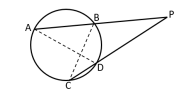• Instruction includes relating the Intersecting Secants Theorem to the Tangent-Secant Theorem and the Tangent-Tangent Theorem. Students can build the understanding that the Tangent-Secant Theorem and the Tangent-Tangent Theorem are specific cases of the Intersecting Secants Theorem.
• For example, to prove the Tangent-Secant Theorem, using the above circle, students can move points C and D toward one another until they meet at a tangent point, T , creating the tangent segment TP. Students then can determine that PA ⋅ PB = PT ⋅ PT which is equivalent to PA ⋅ PB = PT2
• For example, to prove the Tangent-Tangent Theorem, using the above circle, students can move points C and D toward one another until they meet at a tangent point, T, creating the tangent segment TP. Likewise, students can move points A and B towards one another until they meet at a tangent point, SP, creating the tangent segment TP. Students then can determine that PA ⋅ PB = PT ⋅ PT which is equivalent to PA . PB = PT2
• Instruction includes the connection to properties of perpendicular bisectors to prove, and understand, the relationship between a chord and the diameter of the circle that is perpendicular to the chord.
• Instruction includes the connection to the Pythagorean Theorem to prove, and understand, the relationship between a tangent segment, the radius of the circle to the point of tangency and the line segment from the center of the circle to the external point.
• For example, given the circle below, students can use the Pythagorean Theorem to show that $t$2 + $r$2 = $l$2. Students can then rearrange the formula to highlight the length of the tangent segment in terms of the length of the radius and the distance between the center and the external point.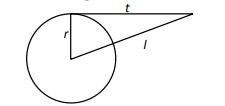### Common Misconceptions or Errors

• Students may confuse the exterior portion of a secant and the whole secant when determining lengths or products of lengths.

• Circle C is shown below with various lines and line segments. PS and PT are tangent to Circle C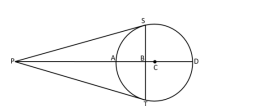• Part A. Write a statement of equality that involves the length of a tangent and the length of a secant.
• Part B. Write a statement of equality that involves the lengths of two tangents.
• Part C. Write a statement of equality based on the relationship between a chord and a diameter.
• Part D. Write a statement of equality that involves the length of a tangent, the length of a line from the center to the external point and the length of a radius.
• Part E. Compare your statements from Parts A, B, C and D with a partner.

• In Circle A, AE = DE, FE= 6 inches and GE= 10 inches. What is the length of the radius of Circle A?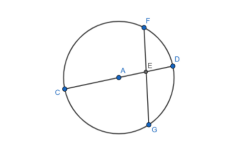### Instructional Items

Instructional Item 1
• In Circle A, DE and BC intersect at point F. FE = 1.3 units, BF = 1.9 units, FD = $x$ + 1.3 units and CF = $x$ units. Find the value of $x$.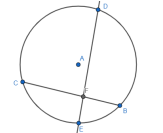*The strategies, tasks and items included in the B1G-M are examples and should not be considered comprehensive.

## Related Courses

This benchmark is part of these courses.
1200400: Foundational Skills in Mathematics 9-12 (Specifically in versions: 2014 - 2015, 2015 - 2022, 2022 and beyond (current))
1206310: Geometry (Specifically in versions: 2014 - 2015, 2015 - 2022, 2022 and beyond (current))
1206320: Geometry Honors (Specifically in versions: 2014 - 2015, 2015 - 2022, 2022 and beyond (current))
1206315: Geometry for Credit Recovery (Specifically in versions: 2014 - 2015, 2015 - 2022, 2022 and beyond (current))
7912065: Access Geometry (Specifically in versions: 2015 - 2022, 2022 and beyond (current))

## Related Access Points

Alternate version of this benchmark for students with significant cognitive disabilities.
MA.912.GR.6.AP.1: Identify and describe the relationship involving the length of a secant, tangent, segment or chord in a given circle.

## Related Resources

Vetted resources educators can use to teach the concepts and skills in this benchmark.

## Formative Assessment

Students are asked to draw a circle, a tangent to the circle, and a radius to the point of tangency. Students are then asked to describe the relationship between the radius and the tangent line.

Type: Formative Assessment

## Lesson Plan

Seeking Circle Segments:

Students will start this lesson with a "Pictionary" game to review circle vocabulary terms. They will then use computers and GeoGebra to discover the relationships between portions of segments that intersect in the interior of the circle, and exterior to the circle. They will wrap up the lesson in a class discussion and consensus on rules (formulas).

Type: Lesson Plan